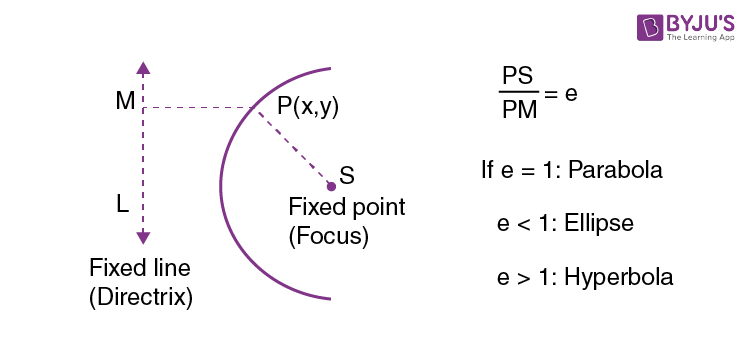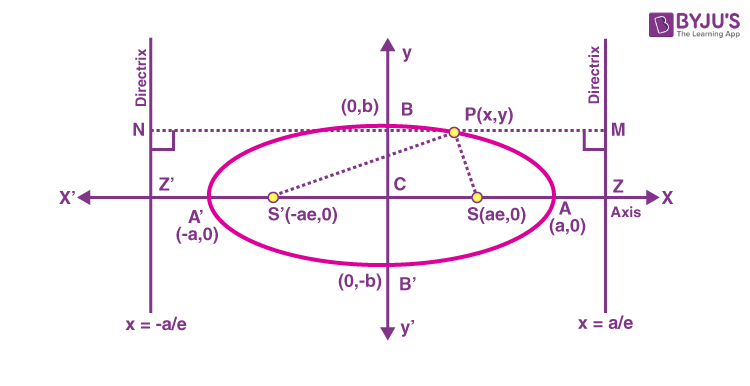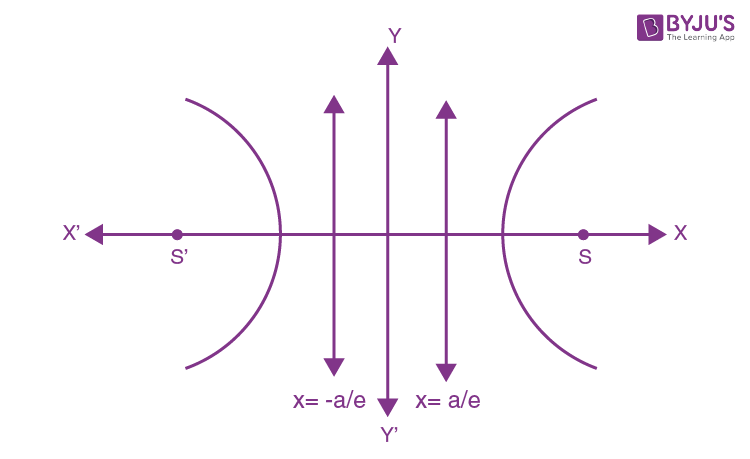Win up to 100% scholarship on Aakash BYJU'S JEE/NEET courses with ABNAT Win up to 100% scholarship on Aakash BYJU'S JEE/NEET courses with ABNAT

# Conic Sections Standard Form

A conic (section) is the locus of a point moving in a plane, such that its distance from a fixed point (focus) is in a constant ratio to its perpendicular distance from a fixed line, i.e., directrix. This constant ratio is called the eccentricity of the conic.

The eccentricity of a circle is zero. It shows how “un-circular” a curve is. Higher the eccentricity, the lower curved it is.Terminology:

Axis of conic: Line passing through focus, perpendicular to the directrix.

Vertex: Point of the intersection of conic and axis.

Chord: Line segment joining any 2 points on the conic.

Double ordinate: Chord perpendicular to the axis

Latus rectum: Double ordinate passing through focus.

## Standard Parabola

 Standard Equation Directrix Focus Length of Latus Rectum Vertex y2 = 4ax x = – a S : (a, 0) 4a (0, 0) y2 = – 4ax x = a (- a, 0) 4a (0, 0) x2 = 4ay y = – a (0, +a) 4a (0, 0) x2 = – 4ay y = a (0, -a) 4a (0, 0)

Important results of a parabola

1. 4 x distance between vertex and focus = Latus rectum = 4a.

2. 2 x distance between directrix and focus = Latus rectum = 2(2a).

3. Point of intersection of the axis and directrix, and the focus is bisected by the vertex.

4. Focus is the midpoint of the Latus rectum.

5. (Distance of any point on the parabola from the axis)2 = (LR) (Distance of the same point from tangent at the vertex)

## Ellipse

It is a locus of a point which moves such that the ratio of its distance from a fixed point (focus) to its distance from a fixed line (directrix) is always constant and less than 1, i.e., 0 < e < 1.Ellipse with a horizontal major axis

$$\begin{array}{l}\frac{{{x}^{2}}}{{{a}^{2}}}+\frac{{{y}^{2}}}{{{b}^{2}}}=1;b<a\end{array}$$

Focus : There are 2 focii: (ae, 0) and (-ae, 0)

Directrix: These foci have corresponding directrices as x = +a/e and x = -a/e, respectively.

$$\begin{array}{l}{{e}^{2}}=1-\frac{{{b}^{2}}}{{{a}^{2}}}\end{array}$$

Axes:

xx’ : Major Axis: Length: 2a

yy’ : Minor axis: length : 2b

Vertex : (a, 0) & (-a, 0)

Centre : (0, 0)

Latus rectum:

$$\begin{array}{l}y=\pm \frac{b^2}{R}\end{array}$$
$$\begin{array}{l}\left( solve\,x=\pm \,ae\,with\,\frac{{{x}^{2}}}{{{a}^{2}}}+\frac{{{y}^{2}}}{{{b}^{2}}}=1 \right)\end{array}$$

Length of Latus rectum:

$$\begin{array}{l}y=\frac{2b^2}{a}\end{array}$$

Ellipse with the vertical major axis

$$\begin{array}{l}\frac{{{x}^{2}}}{{{a}^{2}}}+\frac{{{y}^{2}}}{{{b}^{2}}}=1;b>a\end{array}$$
$$\begin{array}{l}\Rightarrow {{e}^{2}}=1-\frac{{{a}^{2}}}{{{b}^{2}}}\end{array}$$

Length of major axis: 2b

Length of minor axis: 2a

Focii : (0, be) & (0, -be)

Directrices: x = b/e & x = -b/e

Latus rectum: y = + be

Length of Latus rectum:

$$\begin{array}{l}\frac{2{{a}^{2}}}{b}\end{array}$$

Important results

1. $$\begin{array}{l}e=\sqrt{1-\frac{{{(semi\ minor\ axis)}^{2}}}{{{(semi\ major\ axis)}^{2}}}}\end{array}$$
2. $$\begin{array}{l}Length\ of\ Latus\ Rectum=\frac{2(semi\ minor\ axis)^2}{(semi\ minor\ axis)}\end{array}$$
3. Distance between 2 directices:
$$\begin{array}{l}\frac{Major\,axis}{eccentricity}\end{array}$$
4. Distance between 2 foci: (major axis) × eccentricity
5. Distance between focus and directrix:
$$\begin{array}{l}\frac{a}{e}-ae\,\,or\,\,\frac{b}{e}-be\end{array}$$

## Hyperbola

It is the locus of a point which moves such that the ratio of its distance from a fixed point (focus) to its distance from a fixed line (directrix) is always constant and greater than 1.

e > 1Standard hyperbola:

Equation:

$$\begin{array}{l}\frac{{{x}^{2}}}{{{a}^{2}}}-\frac{{{y}^{2}}}{{{b}^{2}}}=1\end{array}$$

Focus: There are 2 foci (ae, 0) and (-ae, 0)

Directrix: The foci have corresponding directrices as x = +a/e and x = −a/e, respectively.

$$\begin{array}{l}{{e}^{2}}=1+\frac{{{b}^{2}}}{{{a}^{2}}};{{b}^{2}}={{a}^{2}}({{e}^{2}}-1)\end{array}$$

Axis: xx’ : Transverse axis ; Length : 2a

yy’ : Conjugate axis ; Length : 2b (Hypothetical)

Vertex : (0, 0) and (-a, 0)

Centre: (0, 0)

Latus rectum: x = +ae

Length of latus rectum:

$$\begin{array}{l}\frac{2{{b}^{2}}}{a}\end{array}$$

Position of point at hyperbola

$$\begin{array}{l}\frac{{{x}^{2}}}{{{a}^{2}}}-\frac{{{y}^{2}}}{{{b}^{2}}}=1\end{array}$$
$$\begin{array}{l}\text{Let}\ S=\frac{{{x}^{2}}}{{{a}^{2}}}-\frac{{{y}^{2}}}{{{b}^{2}}}-1\end{array}$$

and

$$\begin{array}{l}{{S}_{1}}=\frac{{{x}_{1}}^{2}}{{{a}^{2}}}-\frac{{{y}_{1}}^{2}}{{{b}^{2}}}-1\end{array}$$

If S1 > 0, point C lies inside the hyperbola

S1 = 0 point B lies on the hyperbola

S1 < 0 point A lies outside the hyperbola.

In Short:

Equations of some of the conic sections when the centre is the origin or any given point, say (h, k), are as follows:

 Conic section Centre at origin Centre is (h, k) Circle x2 + y2 = r2; r is the radius (x – h)2 + (y – k)2 = r2; r is the radius Hyperbola (x2/a2) – (y2/b2) = 1 (x – h)2/a2 – (y – k)2/b2 = 1 Ellipse (x2/a2) + (y2/b2) = 1 (x – h)2/a2 + (y – k)2/b2 = 1

## Conic Section Videos

### Visualising Conic Sections### Degenerate & Non-Degenerate Conics### Conic Sections### Solved Examples

Example 1: Find the equation of a conic whose focus is at (1, 0) and whose directrix is 2x + 5y + 1 = 0. Also, e = 1/√2.

Solution:

$$\begin{array}{l}\frac{1}{\sqrt{2}}=\frac{\sqrt{{{(h-1)}^{2}}+{{k}^{2}}}}{\frac{12h+5k+11}{\sqrt{29}}}\end{array}$$
$$\begin{array}{l}\frac{12x+5y+11}{\sqrt{58}}=\sqrt{{{(x-1)}^{2}}+{{y}^{2}}}\end{array}$$

Squaring both sides, we get

4x2 + 25y2 + 1 + 20xy + 10y + 4x

Which is the required equation.

Example 2: If extreme points of LR are (11/2, 6) and (13/2, 4), find the equation of the parabola.

Solution:

Midpoint of LR = focus = (6, 5)

Now, 4a = 2 or a = ½

The equation of parabolas is:

(y – 5)2 = 2(x – 5.5) and (y – 5)2 = – 2 (x – 6.5)

## Conic Section – JEE Advanced PY Questions## Most Important JEE Advanced Questions from Conic SectionsQ1

### What do you mean by a conic section?

A conic section is a curve obtained by the intersection of the surface of a cone with a plane.

Q2

### Name three types of conic sections.

The three types of conic sections are the hyperbola, parabola, and ellipse.

Q3

### Give two applications of the conic section.

Conic sections are used by architectural engineers and architects.

Q4

### What do you mean by the eccentricity of a conic section?

The eccentricity of a conic section is defined as the distance from any point to its focus, divided by the perpendicular distance from that point to its nearest directrix.

Test your Knowledge on conic sections